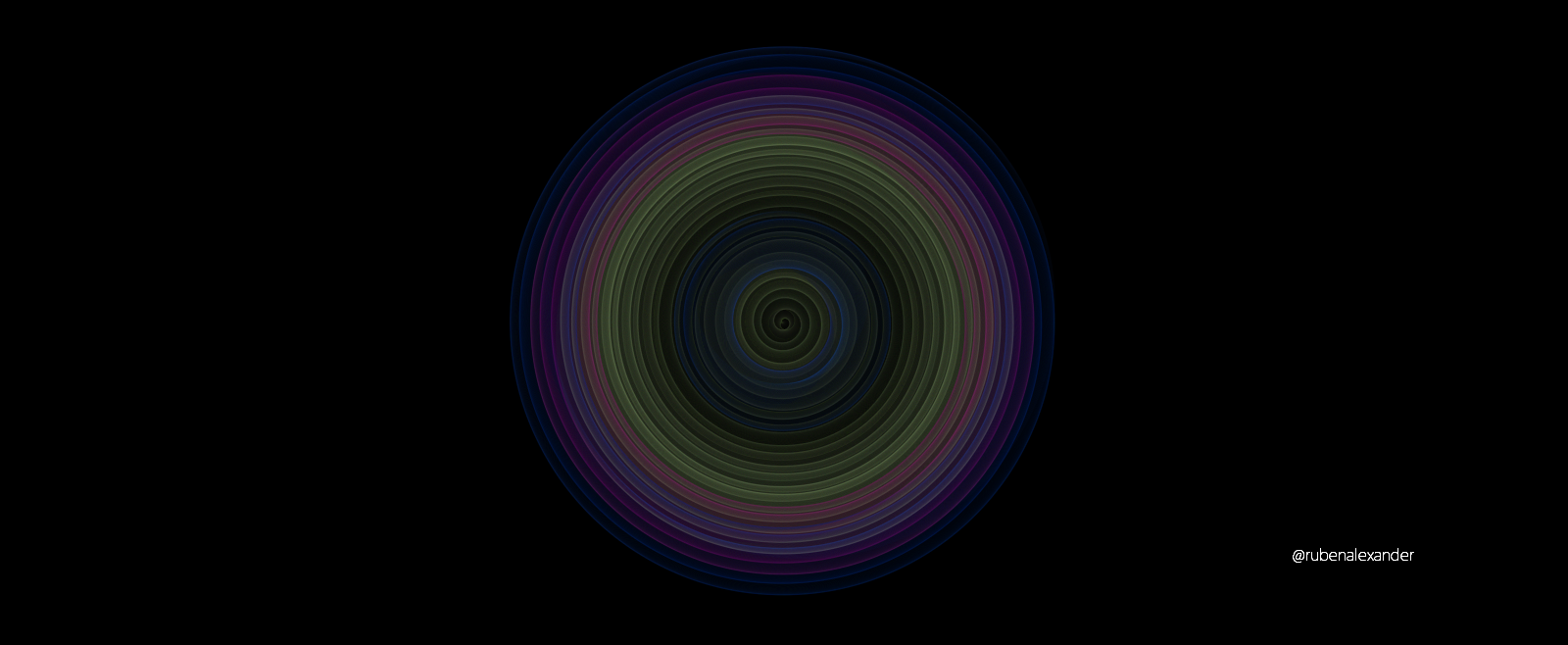# Code Art 01122020I went through old code and found a neat lerp algorithm. Two circles rotate and a third chases the previous angle between the two moving circles. The path of the third is drawn with very low (I believe it is 5) opacity.``````let v1,v2,v3,v4;var size=1;var rot1=30/100;var rot2=40/200;function setup() {createCanvas(windowWidth, windowHeight);background(0);
noFill();
v1 = createVector(-20, 20);
v2 = createVector(20, 20);
}function draw() {var r = 0;var g = 255;var b = 100;
r+=5;

var step=.05;

translate(width/2,height/2);

v1.rotate(rot1);
v2.rotate(rot2);
v3 = p5.Vector.lerp(v1, v2, step);

stroke((5*second())%255,(6*second())%255,(4*second())%255,5);
ellipse(v3.x,v3.y,size,size);
size+=.1;
}function roll(n){return floor(random(n));}
https://www.openprocessing.org/user/168676#sketches``````

``` (adsbygoogle = window.adsbygoogle || []).push({}); Comments 2#rubenalexanderTwitter link: https://twitter.com/rub3al3xand3r/status/1216565719120392194 13.01.2020 03:41 0#blazingnice one keep doing on your great work :) 13.01.2020 14:28 1 ```
``` ```
``` ```
``` Gold Voice social club © GoldVoice.club | Tags Drop image here… … (function(i,s,o,g,r,a,m){i['GoogleAnalyticsObject']=r;i[r]=i[r]||function(){(i[r].q=i[r].q||[]).push(arguments)},i[r].l=1*new Date();a=s.createElement(o),m=s.getElementsByTagName(o);a.async=1;a.src=g;m.parentNode.insertBefore(a,m)})(window,document,'script','https://www.google-analytics.com/analytics.js','ga');ga('create', 'UA-104889202-1', 'auto');ga('send', 'pageview'); ```